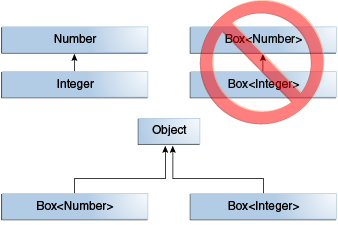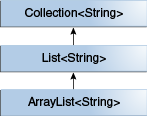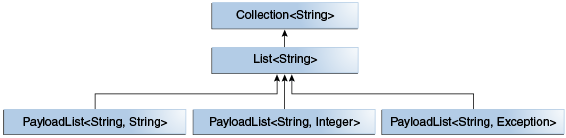# Java 之路 (十五) -- 泛型上（泛型类、泛型方法、有界类型参数、泛型与继承、类型推断）

Thinking in Java 中关于泛型的讲解篇幅实在过长，前后尝试阅读这一章，但总是觉得找不到要点，很迷。于是放弃 Thinking in Java 泛型一章的阅读，转而官方教程，本章可以算作官方教程的中文版。

The Java™ Tutorials - Generics

# 1.为什么使用泛型

• 在编译时进行更强大的类型检查。
Java编译器将强类型检查应用于通用代码，并在代码违反类型安全时发出错误。修复编译时错误比修复运行时错误更容易，后者很难发现错误源头。

• 消除转型

以下没有泛型的代码片段需要强制转换：

List list = new ArrayList();
String s = (String)list.get(0);

使用泛型时，不需要类型转换：

List <String> list = new ArrayList<String>();
String s = list.get(0); //没有转型
• 使程序员能够实现通用算法。
通过使用泛型，程序员可以实现通用算法，这些算法可以处理不同类型的集合，可以自定义，并且类型安全且易于阅读。

# 2. 泛型类

## 2.1 简单的 Box 类

public class Box {
private Object object;

public void set(Object object) { this.object = object; }
public Object get() { return object; }
}

## 2.2 Box 类的泛型版本

class name<T1, T2, ..., Tn> { /* ... */ }

public class Box<T> {
// T stands for "Type"
private T t;

public void set(T t) { this.t = t; }
public T get() { return t; }
}

interface Box<T> { /*...*/}

## 2.3 类型参数命名约定

• E - Element（Java Collections Framework广泛使用）
• K - key
• N - number
• T - 类（类型）
• V - value
• S，U，V等 - 第2，第3，第4类型

## 2.4 调用和实例化泛型类

 Box<Integer> integerBox = new Box<Integer>();

//在 Java SE 7 及更高版本中，只要编译期可以从上下文中确定或推断类型参数，就可以用一组空的类型参数“<>” 替换调用泛型类的构造函数所需的类型参数
//如下：
Box<Integer> integerBox = new Box<>();

## 2.5 多种类型参数

public interface Pair<K, V> {
public K getKey();
public V getValue();
}

public class OrderedPair<K, V> implements Pair<K, V> {

private K key;
private V value;

public OrderedPair(K key, V value) {
this.key = key;
this.value = value;
}

public K getKey()   { return key; }
public V getValue() { return value; }
}

Pair<String，Integer> p1 = new OrderedPair<String，Integer>("Even"，8);
Pair<String，String> p2 = new OrderedPair <String，String>("hello","world");

//或如下
Pair<String，Integer> p1 = new OrderedPair<>("Even"，8);
Pair<String，String> p2 = new OrderedPair <>("hello","world");

## 2.6 参数化类型

OrderedPair<String, Box<Integer>>> p = new OrderedPair<>("primes"，new Box<Integer>(...));

## 2.7 “原生”类型

public class Box<T> {
public void set(T t) { /* ... */ }
// ...
}

Box <Integer> intBox = new Box<>();

Box rawBox = new Box();

Box 是泛型 Box<T> 的原生类型。

Box <String> stringBox = new Box<>();
Box rawBox = stringBox;  // 这是没问题的

 Box rawBox = new Box();  // rawBox是Box<T>的原始类型
Box <Integer> intBox = rawBox;  //warning: unchecked conversion

Box <String> stringBox = new Box<>();
Box rawBox = stringBox;
rawBox.set(8);  //warning: unchecked invocation to set(T)

# 3. 泛型方法

[权限修饰词] <T1,T2,...,Tn> methods(/*...*/) {/*...*/}

public class Util {
public static <K, V> boolean compare(Pair<K, V> p1, Pair<K, V> p2) {
return p1.getKey().equals(p2.getKey()) &&
p1.getValue().equals(p2.getValue());
}
}

public class Pair<K, V> {

private K key;
private V value;

public Pair(K key, V value) {
this.key = key;
this.value = value;
}

public void setKey(K key) { this.key = key; }
public void setValue(V value) { this.value = value; }
public K getKey()   { return key; }
public V getValue() { return value; }
}

Util 类包含一个泛型方法 compare，用以比较两个 Pair 对象。

Pair <Integer,String> p1 = new Pair<>(1,"apple");
Pair <Integer,String> p2 = new Pair<>(2,"pear");
boolean same = Util.<Integer,String> compare(p1,p2);

Pair <Integer,String> p1 = new Pair<>(1,"apple");
Pair <Integer,String> p2 = new Pair<>(2,"pear");
boolean same = Util.compare(p1,p2);

# 4. 有界类型参数

<T extends SomeType> 

public class Box<T> {

private T t;

public void set(T t) {
this.t = t;
}

public T get() {
return t;
}

public <U extends Number> void inspect(U u){
System.out.println("T: " + t.getClass().getName());
System.out.println("U: " + u.getClass().getName());
}

public static void main(String[] args) {
Box<Integer> integerBox = new Box<Integer>();
integerBox.set(new Integer(10));
integerBox.inspect("some text"); // error: this is still String!
}
}

public class NaturalNumber<T extends Integer> {

private T n;

public NaturalNumber(T n)  { this.n = n; }

public boolean isEven() {
return n.intValue() % 2 == 0;
}

// ...
}

isEven() 方法通过 n 调用 Integer 类中定义的 intValue 方法。

## 4.1 多个边界

<T extends B1 & B2 & B3>

Class A { /* ... */ }
interface B { /* ... */ }
interface C { /* ... */ }

class D <T extends A & B & C> { /* ... */ }

## 4.2 泛型方法与有界类型参数

public static <T> int countGreaterThan(T[] anArray, T elem) {
int count = 0;
for (T e : anArray)
if (e > elem)  // compiler error
++count;
return count;
}

public interface Comparable<T> {
public int compareTo(T o);
}

public static <T extends Comparable<T>> int countGreaterThan(T[] anArray, T elem) {
int count = 0;
for (T e : anArray)
if (e.compareTo(elem) > 0)
++count;
return count;
}

# 5. 泛型与继承

Object someObject = new Object();
Integer someInteger = new Integer(10);
someObject = someInteger;  // Object 是 Integer 的父类

public void someMethod(Number n) { /* ... */ }

someMethod(new Integer(10));   // OK
someMethod(new Double(10.1));   // OK

Box<Number> box = new Box<Number>();
box.add(new Double(10.1));  // OK

public void boxTest(Box<Number> n) { /* ... */ }

**你可能会因为它可以接受一个类型为 Box<Number> 的参数，按照上面的结论，就以为向其传递 Box<Integer> 或者 Box<Double> ？这里需要强调，后者是不能传递的。因为 Box<Integer>Box<Double> 并不是 Box<Number> 的子类型。## 5.1 泛型类和子类型interface PayloadList<E,P> extends List<E> {
...
}

• PayloadList <字符串，字符串>
• PayloadList <字符串，整数>
• PayloadList <字符串，异常># 6. 类型推断

## 6.1 类型推断与泛型方法

boolean same = Util.<Integer,String> compare(p1,p2);//指定类型
boolean same = Util.compare(p1,p2);//不指定类型，Java 编译期会自动推断类型参数是Integer 和 String

## 6.2 类型推断和泛型类的实例化

Map<String, List<String>> myMap = new HashMap<String, List<String>>();

Map<String, List<String>> myMap = new HashMap<>();

## 6.3 类型推断 与 泛型/非泛型类的泛型构造方法

class MyClass<X> {
<T> MyClass(T t) {
// ...
}
}

new MyClass<Integer>("");

Java SE7之前，编译期能够推断泛型构造参数的类型参数。Java SE7 之后，使用 <> 使编译期推断正在实例化的泛型类的类型参数：

MyClass<Integer> myObject = new MyClass<>("");

## 6.4 目标类型

Java编译器利用目标类型来推断泛型方法调用的类型参数。 表达式的目标类型是Java编译器所期望的数据类型，具体取决于表达式的显示位置。 考虑方法Collections.emptyList ，声明如下：

static <T> List<T> emptyList();

List<String> listOne = Collections.emptyList();

List<String> listOne = Collections.<String>emptyList();

void processStringList(List<String> stringList) {
// process stringList
}

processStringList(Collections.emptyList());©️2019 CSDN 皮肤主题: 编程工作室 设计师: CSDN官方博客